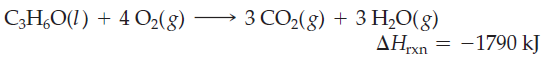×
Get Full Access to Introductory Chemistry - 5 Edition - Chapter 8 - Problem 75p
Get Full Access to Introductory Chemistry - 5 Edition - Chapter 8 - Problem 75p

×

Octane (C8H18) is a component of gasoline that burns according to the equation: WhatISBN: 9780321910295 34

Solution for problem 75P Chapter 8

Introductory Chemistry | 5th Edition

• Textbook Solutions
• 2901 Step-by-step solutions solved by professors and subject experts
• Get 24/7 help from StudySoup virtual teaching assistantsIntroductory Chemistry | 5th Edition

4 5 1 271 Reviews
15
4
Problem 75P

Octane (C8H18) is a component of gasoline that burns according to the equation:What mass of octane (in g) is required to produce 1.55103 kJ of heat?

Step-by-Step Solution:
Step 1 of 3

Solution 75PFrom the equation given above it is clear that 1 mole of C8H18 releases -5074KJ of energy

The amount of C8H18 that will release 1.55 × 103kJ of heat will be=-1.55 ×103kJ=0.30moles

Molecular mass of C8H18=8 ×12+18 ×1=114g

Weight in grams of  C8H18=Number of moles ×Molecular mass=0.30 ×114=34.2grams

Thus 34.2 grams of octane will release 1.55 × 103kJ of energy

Step 2 of 3

Step 3 of 3

ISBN: 9780321910295

Since the solution to 75P from 8 chapter was answered, more than 865 students have viewed the full step-by-step answer. The answer to “?Octane (C8H18) is a component of gasoline that burns according to the equation: What mass of octane (in g) is required to produce 1.55 103 kJ of heat?” is broken down into a number of easy to follow steps, and 28 words. This full solution covers the following key subjects: octane, heat, component, equation, gasoline. This expansive textbook survival guide covers 19 chapters, and 2046 solutions. This textbook survival guide was created for the textbook: Introductory Chemistry, edition: 5. Introductory Chemistry was written by and is associated to the ISBN: 9780321910295. The full step-by-step solution to problem: 75P from chapter: 8 was answered by , our top Chemistry solution expert on 05/06/17, 06:45PM.

Unlock Textbook Solution